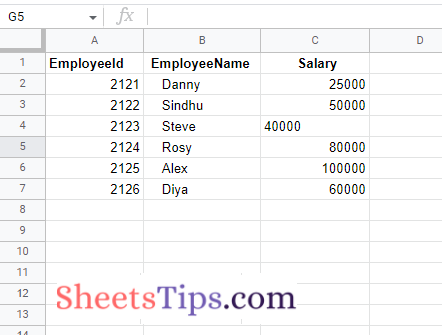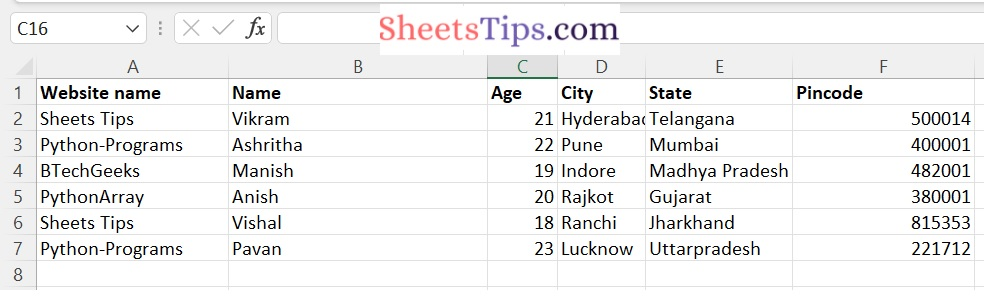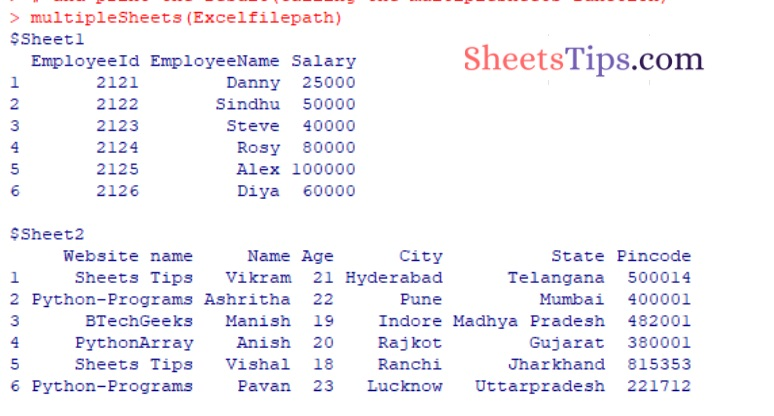# How to read a XLSX file with Multiple Sheets in R?

## How to read a XLSX file with Multiple Sheets in R?

To read XLSX files with several sheets, R has a number of external packages.

This can be done using the following ways:

• Using the rio package.
• Using the openxlsx Package.

Here we now work on the following Excel spreadsheet shown in the image below:

SampleExcelFile:

sheet1:sheet2:## Reading an XLSX File with Multiple Sheets Using R

Below are the ways to read an XLSX File with Multiple Sheets in R:

### Method #1: Using the readxl package.

The readxl package in R is used to import and read Excel workbooks, allowing you to quickly work with and modify.xslsx sheets. The following syntax can be used to install and load it into the R working environment.

Installation:

`install.packages("readxl")`

### Functions Used:

1)lappy() method:

The lapply() method in R is used to apply a user-defined or pre-defined function to a set of components included within an R list or data frame. The method lapply() returns an object with the same length as the supplied/given object.

Syntax:

`lapply( object, FUNCTION)`

Parameters

object: It is the object to which the function will be applied.

FUNCTION: It is the function that will be applied to different components of the object.

The FUNCTION here is the read_excel method of this package store, which reads the contents of the specified sheet name into a tibble, which is a tabular-like structure used to store data in fixed rows and columns.

The read_excel method is applied to all sheets of the workbook by the lapply() method.

Syntax:

`read_excel(path, sheet)`

Parameters

path: It is the path of the file given as input.

sheet: It is the sheet name to be read.

The tibble objects given by the read_excel method can be again converted back to a data frame using the lapply method and the function as.data.frame(), which transforms every object into a data frame. Using the built-in R names() method, these data frames can be assigned the respective sheet names for more clarity.

`names (df) <- new-name`

### CODE

Approach:

• Importing readxl package using the library() function
• Create a function say multipleSheets which accepts the filename as an argument and prints the data of the multiple sheets of an excel file.
• Pass the file name as an argument to the excel_sheets function of the readxl library and store it in a variable.
• Get the dataframe of the above excel file using the lapply() function
• Store the names of the dataframes of the sheets using the names() function.
• Print the dataframe values using the print() function
• Give the Excel file path as static input and store it in a variable
• Pass the above given excel file as an argument to the multipleSheets function and print the result (Calling the multipleSheets function).
• The Exit of the Program.

Below is the implementation:

```# Importing readxl package using the library() function
# Create a function say multipleSheets which accepts the filename as an argument
# and prints the data of the multiple sheets of an excel file.
multipleSheets <- function(filename) {

# Pass the file name as an argument to the excel_sheets function of the readxl
# library and store it in a variable.
# Get the dataframe of the above excel file using the lapply() function
datafrme <- lapply(tibble, as.data.frame)

# Store the names of the dataframes of the sheets using the names() function.
names(datafrme) <- xl_sheets

# Print the dataframe values using the print() function
print(datafrme)
}

# Give the Excel file path as static input and store it in a variable
# Pass the above given excel file as an argument to the multipleSheets function
# and print the result(Calling the multipleSheets function)
multipleSheets(Excelfilepath)```

Output:### Method #2: Using the rio package.

The rio package is used to support quick and easy data import and export activities in R. Rio deduces information about the file format itself that can be utilized to conveniently read files.

Installation:

`install.packages("rio")`

### Functions Used:

The R language import() and export() functions identify the data structure of a file extension. The import_list() method loads/imports a list of data frames from a multi-object file, such as an Excel workbook or an R zipped file.

Syntax:

`import_list(file)`

Parameters

file: It is the file name/path of the Excel workbook to work with given as input.

When reading the Excel worksheet output, the column and row names are retained. During the read, the sheet names are also accessible.

### CODE

Approach:

• Give the Excel file path as static input and store it in a variable
• Importing rio package using the library() function
• Pass the above given excel file to the import_list function to list of data frames/data from the given excel file and store it in another variable
• Print the data of the given Excel file using the print() function.
• The Exit of the Program.

Below is the implementation:

```# Give the Excel file path as static input and store it in a variable

# Importing rio package using the library() function
library(rio)

# Pass the above given excel file to the import_list function to list of data frames/data
# from the given excel file and store it in another variable
rslt_data <- import_list(Excelfilepath)

# Print the data of the given Excel file using the print() function
print (rslt_data)```

Output:### Method #3: Using the openxlsx Package.

The R language openxlsx package  is used to create and modify Excel files by providing a high-level interface for reading, writing, and altering the worksheets in the provided workbook. The following syntax can be used to load and install the package into the working space:

Installation:

`install.packages("openxlsx")`

Approach:

• Give the Excel file path as static input and store it in a variable.
• Importing openxlsx package using the library() function.
• Pass the file name/path as an argument to the getSheetNames function of the openxlsx library to get data from the sheets and store it in a variable
• Get the dataframe of the above excel file using the lapply() function
• Store the names of the dataframes of the sheets using the names() function.
• Print the dataframe values using the print() function.
• The Exit of the Program.

Below is the implementation:

```# Give the Excel file path as static input and store it in a variable

# Importing openxlsx package using the library() function
library(openxlsx)

# Pass the file name/path as an argument to the getSheetNames function of the openxlsx
# library to get data from the sheets and store it in a variable
xl_sheets <- openxlsx::getSheetNames(Excelfilepath)
# Get the dataframe of the above excel file using the lapply() function